Courses
Courses for Kids
Free study material
Free LIVE classes
More

# NCERT Solutions for Class 6 Maths Chapter 5 - In HindiLIVE
Join Vedantu’s FREE Mastercalss

## NCERT Solutions for Class 6 Maths Chapter 5 Understanding Elementary Shapes in Hindi PDF Download

Download the Class 6 Maths NCERT Solutions in Hindi medium and English medium as well offered by the leading e-learning platform Vedantu. If you are a student of Class 6, you have reached the right platform. The NCERT Solutions for Class 6 Maths in Hindi provided by us are designed in a simple, straightforward language, which are easy to memorise. You will also be able to download the PDF file for NCERT Solutions for Class 6 Maths in English and Hindi from our website at absolutely free of cost. Subjects like Science, Maths, English,Hindi will become easy to study if you have access to NCERT Solution for Class 6 Science , Maths solutions and solutions of other subjects.

NCERT, which stands for The National Council of Educational Research and Training, is responsible for designing and publishing textbooks for all the classes and subjects. NCERT textbooks covered all the topics and are applicable to the Central Board of Secondary Education (CBSE) and various state boards.

We, at Vedantu, offer free NCERT Solutions in English medium and Hindi medium for all the classes as well. Created by subject matter experts, these NCERT Solutions in Hindi are very helpful to the students of all classes.

Last updated date: 23rd Sep 2023
Total views: 406.8k
Views today: 8.06k

## NCERT Solution for Hindi Chapter 5- प्रारंभिक आकारों को समझना

### प्रश्नावली 5.1

1. रेखाखंड की तुलना केवल देखकर करने से क्या हानि है?

उत्तर: रेखाखंड की तुलना केवल देखकर करने से जब हम लगभग एक ही लंबाई के दो रेखाखंडों की तुलना करते हैं, तो हम अधिक लंबाई के रेखा खंड के बारे में सुनिश्चित नहीं हो सकते। इसलिए, यह उनकी लंबाई के बीच एक मामूली अंतर वाले रेखाखंडों की तुलना करने के लिए एक उपयुक्त तरीका नहीं है। सही माप मिलने की संभावना न के बराबर रहती है। इससे गलती होने की अत्यधिक संभावना रहती है।

2. रेखाखंड की लंबाई मापने के लिए रूलर की अपेक्षा डिवाइडर का प्रयोग करना क्यों अधिक अच्छा है?

उत्तर: रेखाखंड की लंबाई मापने के लिए रूलर की अपेक्षा डिवाइडर का प्रयोग करने से सही माप मिलती है। क्योकि रूलर से मापने के लिए आंखो की स्थिति का सही दिशा मे होना आवश्यक है।

3. कोई रेखाखंड $\mathbf{AB}$ खींचिए। $\mathbf{A}$ और $\mathbf{B}$ के बीच स्थित बिंदु $\mathbf{C}$ लीजिए। $\mathbf{AB},$ $\mathbf{BC}$ और $\mathbf{CA}$ की लंबाई मापिए। क्या $\mathbf{AB}\text{ }=\text{ }\mathbf{AC}\text{ }+\text{ }\mathbf{CB}$ है?
(टिप्पणी: यदि किसी रेखा पर बिंदु $\mathbf{A},\text{ }\mathbf{B},\text{ }\mathbf{C}$ इस प्रकार स्थित हों कि $\mathbf{AC}\text{ }+\text{ }\mathbf{CB}\text{ }=$ $\mathbf{AB}$ है, तो निश्चित रूप से बिंदु $\mathbf{C}$ बिंदु $\mathbf{A}$ और $\mathbf{B}$ के बीच स्थित होता है।)

उत्तर: यदि किसी रेखा पर $A,\text{ }B,\text{ }C$ इस प्रकार स्थित हों कि बिंदु $C$ बिंदु $A$ और $B$ के बीच स्थित हो तो $AB\text{ }=\text{ }AC\text{ }+\text{ }CB$ होगा।

4. एक रेखा पर बिन्दु $\mathbf{A},\text{ }\mathbf{B},\text{ }\mathbf{C}$इस प्रकार स्थित है कि $\mathbf{AB}\text{ }=\text{ }\mathbf{5}$सेमी $\mathbf{BC}\text{ }=\text{ }\mathbf{3}$सेमी और $\mathbf{AC}\text{ }=\text{ }\mathbf{8}$सेमी है। इनमें से कौन सा बिंदु अन्य दोनों बिंदुओं के बीच स्थित है?

उत्तर: दिया है-

$\mathbf{AB}\text{ }=\text{ }\mathbf{5}$,$\mathbf{BC}\text{ }=\text{ }\mathbf{3}$,$\mathbf{AC}\text{ }=\text{ }\mathbf{8}$

यहाँ पर $AB\text{ }+\text{ }BC\text{ }=\text{ }5\text{ }+\text{ }3\text{ }=\text{ }8\text{ }=\text{ }AC$

इसलिए बिंदु $B$ दो बिंदुओं $A$ और $C$ के बीच स्थित है।

5. जाँच कीजिए कि संलग्न आकृति में $\mathbf{D}$ रेखाखंड $\mathbf{AG}$ का मध्य बिंदु है।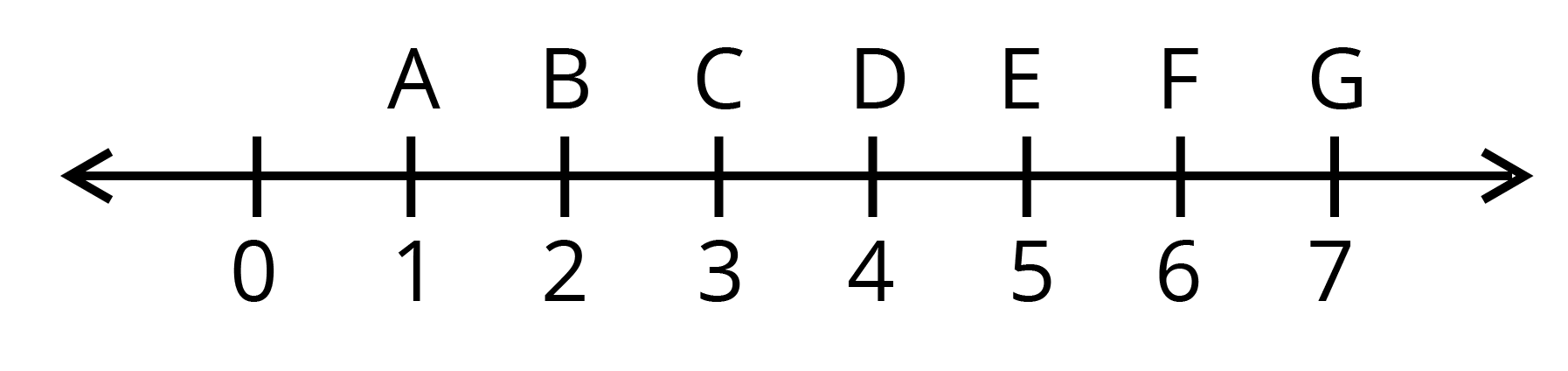उत्तर: चित्रानुसार,

$AG\text{ }=\text{ }7\text{ }\text{ }1\text{ }=\text{ }6$

$AD\text{ }=\text{ }4\text{ }\text{ }1\text{ }=\text{ }3$
चूँकि $3\text{ }=\text{ }6\text{ }\div \text{ }2$

इसलिए, $AD\text{ }=\text{ }AG\text{ }\div \text{ }2$

इससे यह सिद्ध होता है कि बिंदु $D$ रेखाखंड $AG$ का मध्य बिंदु है।

6. $\mathbf{B}$ रेखाखंड $\mathbf{AC}$ का मध्य बिंदु है और $\mathbf{C}$ रेखाखंड $\mathbf{BD}$ का मध्य बिंदु है, जहाँ $\mathbf{A},\text{ }\mathbf{B},\text{ }\mathbf{C}$ और $\mathbf{D}$ एक ही रेखा पर स्थित है। बताइए कि $\mathbf{AB}\text{ }=\text{ }\mathbf{CD}$ क्यों है?

उत्तर:  प्रश्न के अनुसार, एक चित्र बनाने पर,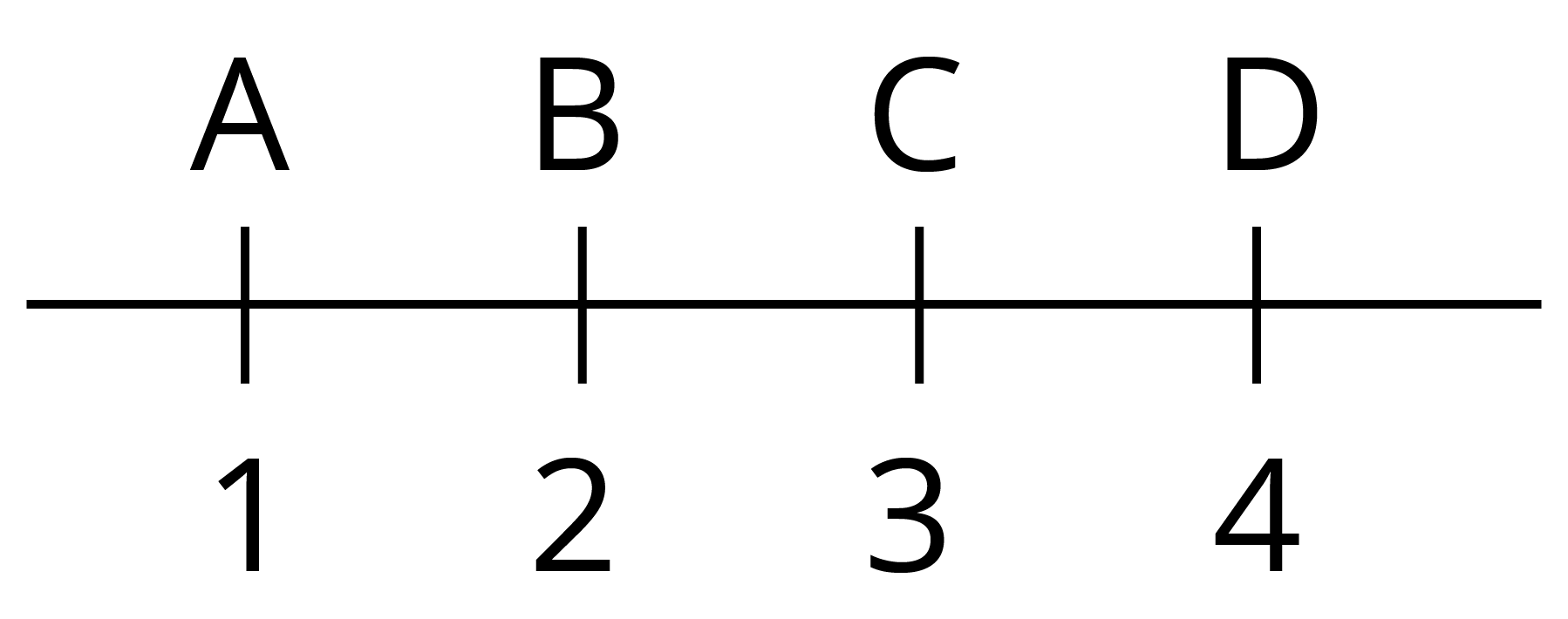$AB\text{ }=\text{ }BC$ ($B$ रेखाखंड $AC$ का मध्य बिंदु है।)
$BC\text{ }=\text{ }CD$ ($C$ रेखाखंड $BD$ का मध्य बिंदु है।)
इसलिए, $AB\text{ }=\text{ }CD$

7. पाँच त्रिभुज खींचिए और उनकी भुजाओं को मापिए। प्रत्येक स्थिति में जाँच कीजिए कि किन्हीं दो भुजाओं की लंबाईओं का योग तीसरी भुजा की लंबाई से सदैव बड़ा है।

उत्तर: हाँ, एक त्रिभुज की दो भुजाओं का योग हमेशा तीसरी भुजा से अधिक है। यह सिद्ध करने का सबसे आसान तरीका है समान लंबाई की दो सींक या माचिस की तीली इस्तेमाल करना। एक तीली को दो बराबर टुकड़ों में तोड़ लीजिए। इन दोनों टुकड़ों की लंबाई का योग बची हुई तीली की लंबाई के बराबर होगा। इन तीनों को मिलाकर आप त्रिभुज बनाने का प्रयास कीजिए। त्रिभुज नहीं बनेगा, क्योंकि दो भुजाओं की लंबाई का योग तीसरी भुजा की लंबाई से अधिक नहीं है।

जैसे-: पाँच त्रिभुज निम्न प्रकार दर्शाए गए हैं,

1. 9+10>15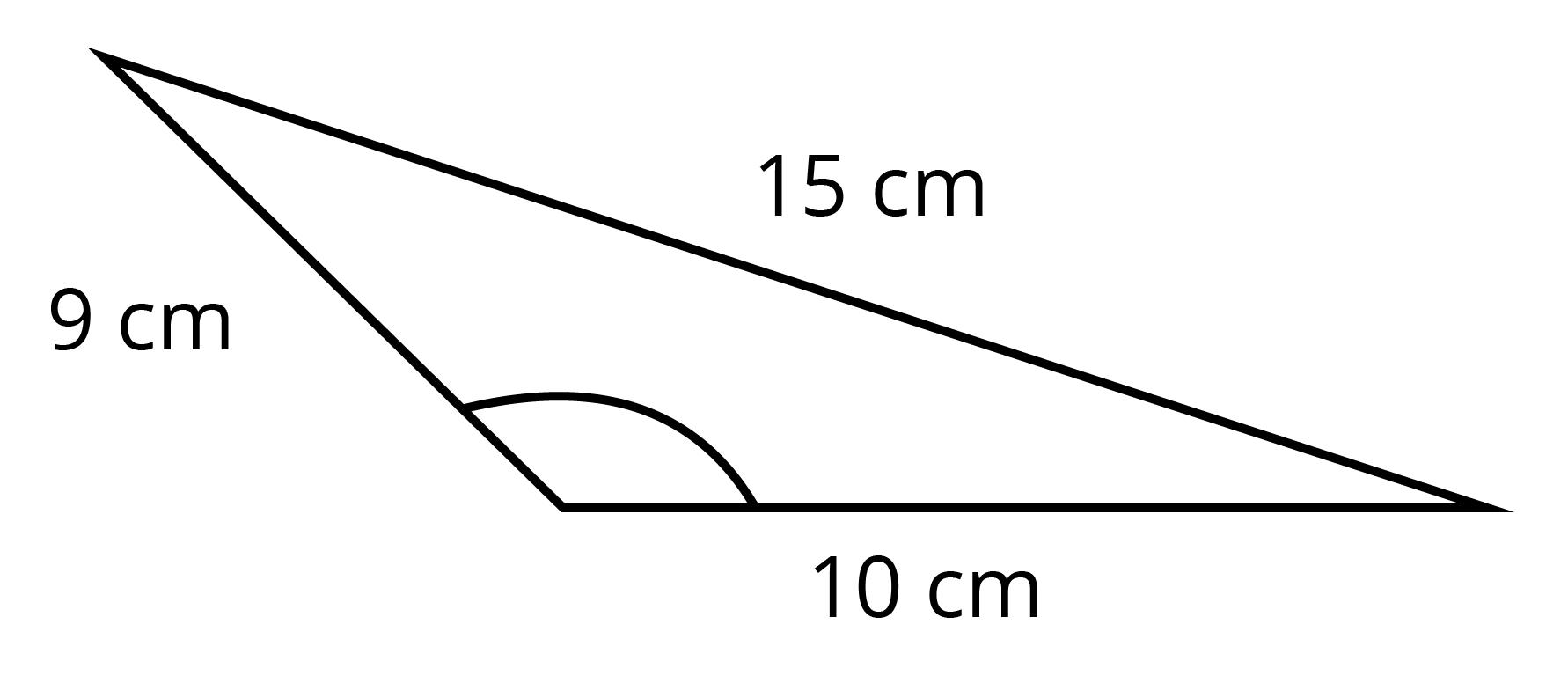1. 5+5>8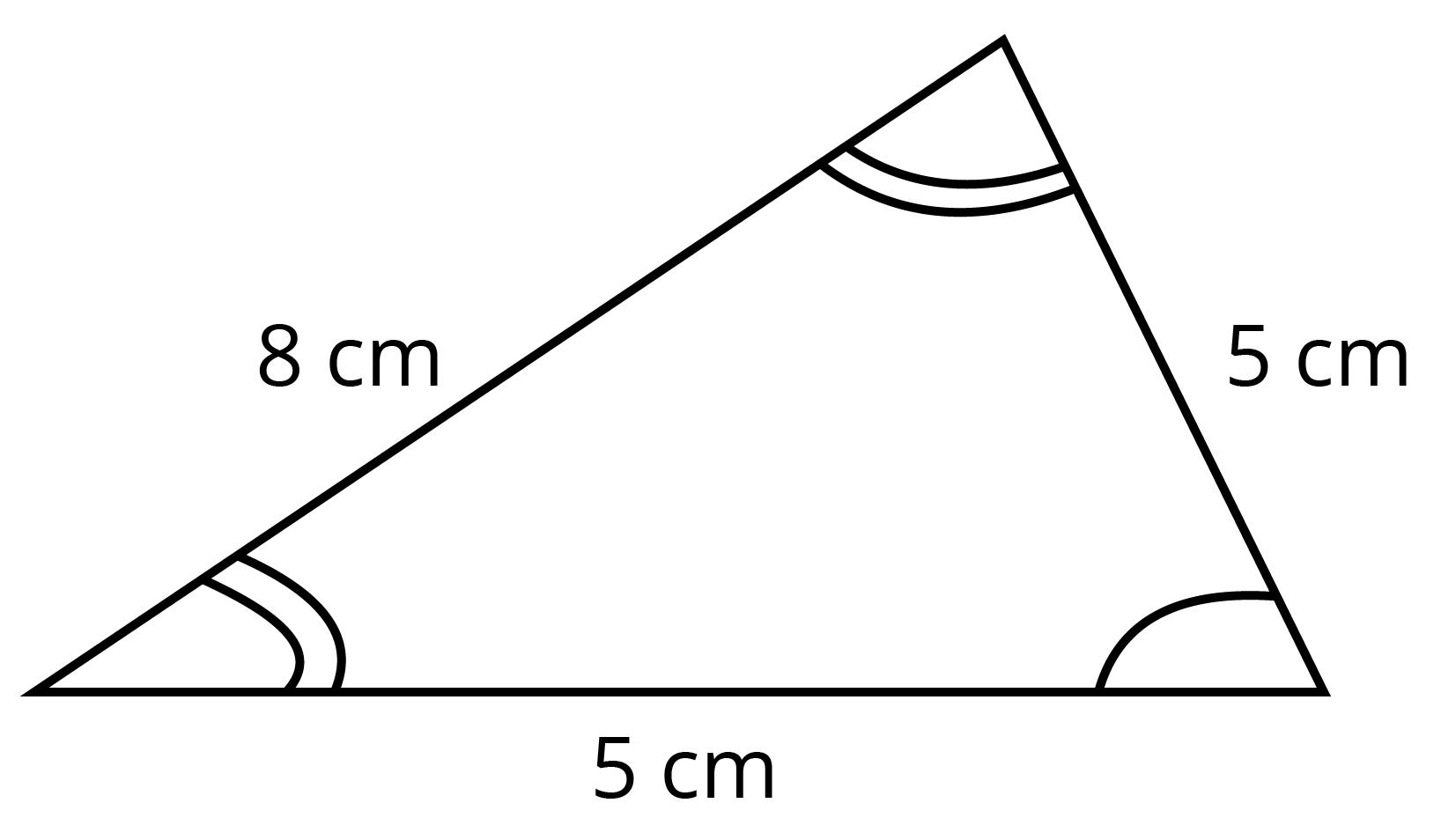1. 5.2+5.2>5.2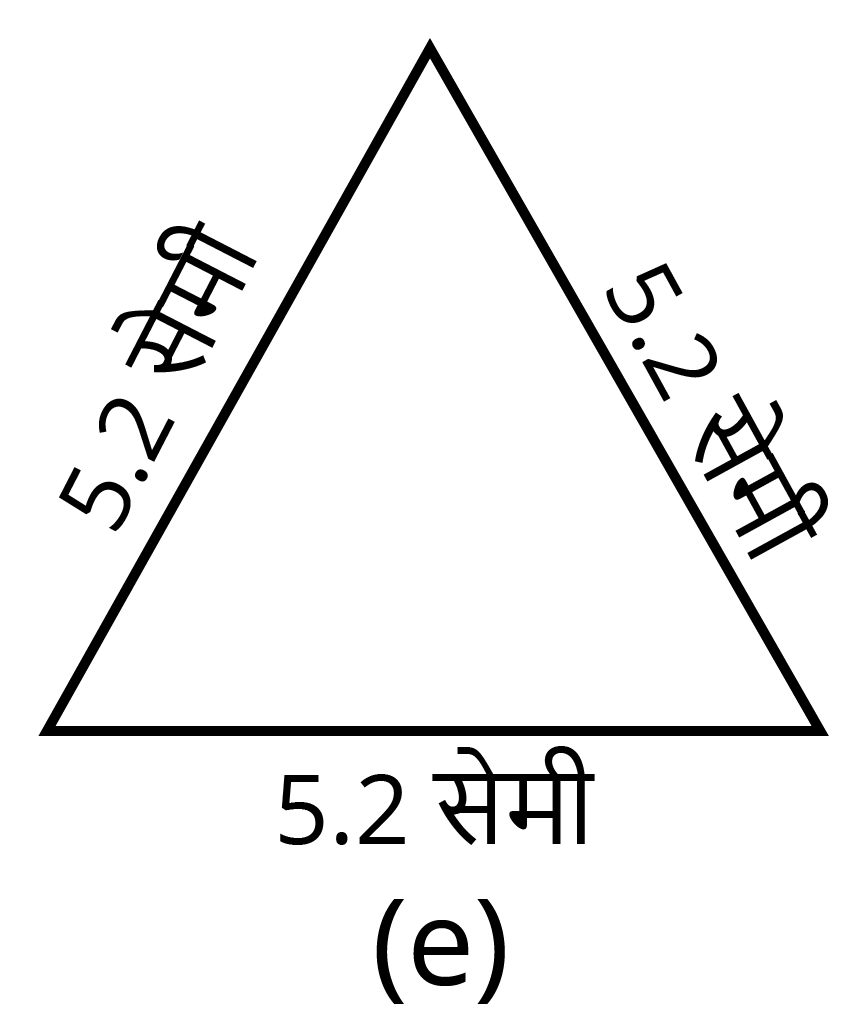1. 10+10>14.14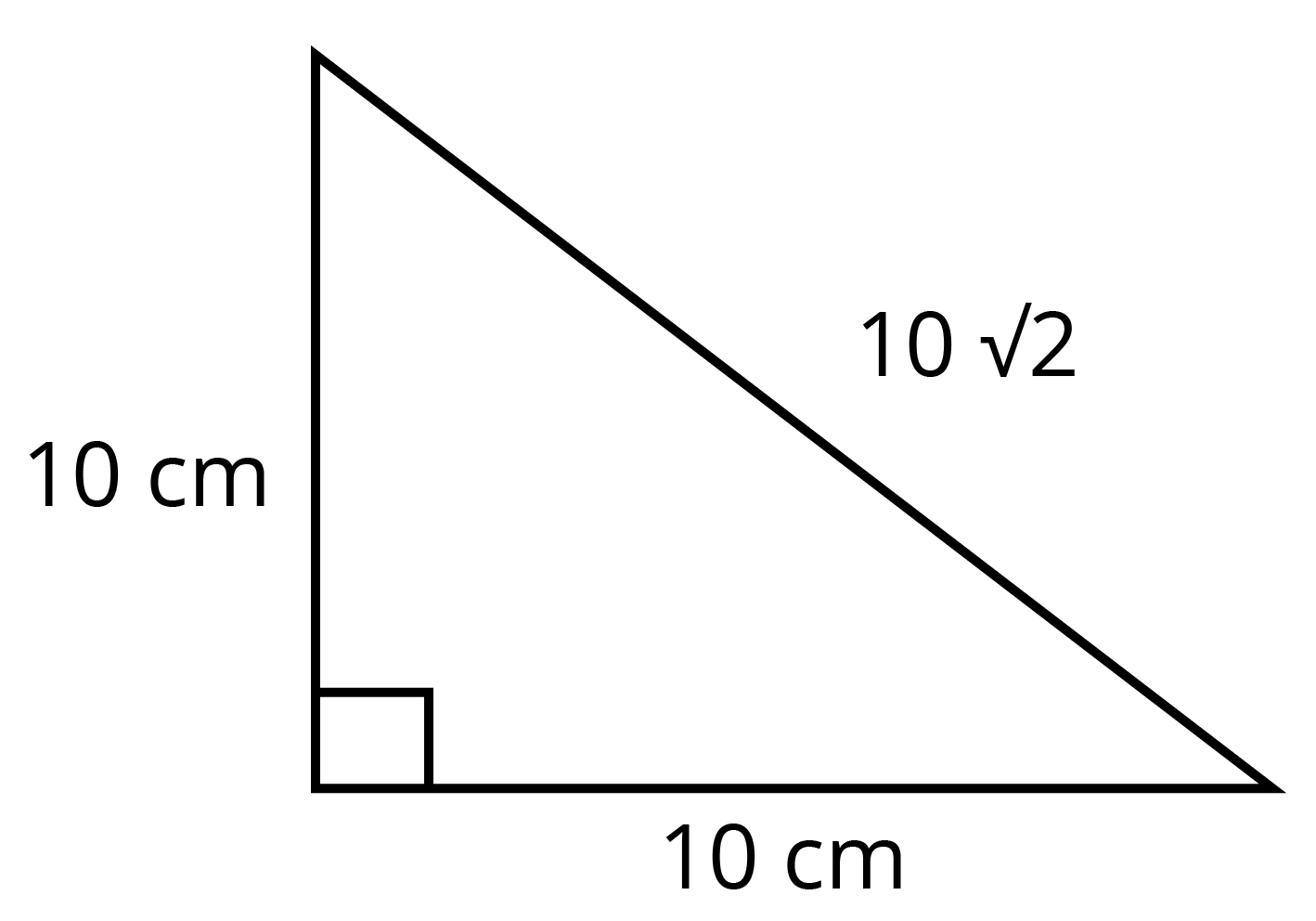1. 8+15>17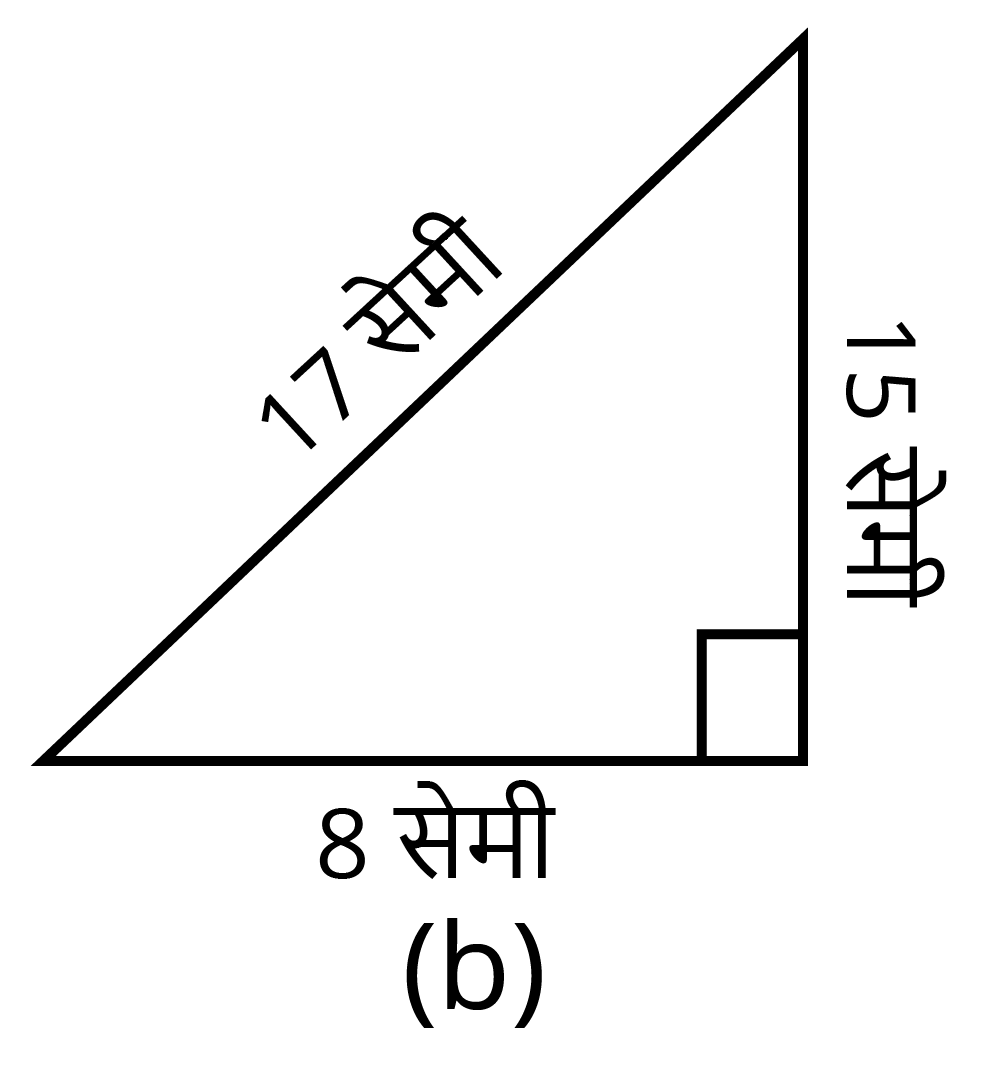### प्रश्नावली 5.2

1. घड़ी की घंटे वाली सुई एक घूर्णन के कितनी भिन्न घूम जाती है, जब वह:

1. 3 से 9 तक पहुँचती है?

2. 4से 7 तक पहुँचती है?

3. 7 से 10 तक पहुँचती है?

4. 12 से 9 तक पहुँचती है?

5. 1 से 10 तक पहुँचती है?

6. 6 से 3 तक पहुँचती है?

उत्तर: घड़ी की घंटे वाली सुई पूरा चक्कर लगाने पर $12$ भाग घूमती है (12 घंटे के बराबर)। इसलिए, एक घंटे में यह पूरे चक्कर का $\frac{1}{12}$ भाग घूमती है।
इसलिए, $9 - 3{\rm{ }} = {\rm{ }}6$ घंटे में यह सुई $\frac{6}{12}=\frac{1}{12}~$ भाग घूमती है।

इसी प्रकार से अन्य प्रश्न हल करेंगे,

$7 - 4{\rm{ }} = {\rm{ }}3$ घंटे में यह सुई $\frac{3}{12}=\frac{1}{4}~$ भाग घूमती है।

$10 - 7{\rm{ }} = {\rm{ }}3$ घंटे में यह सुई $\frac{3}{12}=\frac{1}{4}~$ भाग घूमती है।

$12 - 9{\rm{ }} = {\rm{ }}3$ घंटे में यह सुई $\frac{3}{12}=\frac{1}{4}~$ भाग घूमती है।

$10 - 1{\rm{ }} = {\rm{ }}9$ घंटे में यह सुई $\frac{9}{12}=\frac{3}{4}~$ भाग घूमती है।

$6 - 3{\rm{ }} = {\rm{ }}3$ घंटे में यह सुई $\frac{3}{12}=\frac{1}{4}~$ भाग घूमती है।

अतः,

$\left( a \right)\;\frac{1}{{12}}\;\,\,\,\,\left( b \right)\;\frac{1}{4}\,\,\,\,\,\left( c \right)\,\,\,\,\;\frac{1}{4}\;\,\,\,\,\left( d \right)\;\frac{3}{4}\;\,\,\,\,\left( e \right)\;\frac{5}{6}\;\,\,\,\,\left( f \right)\;\frac{3}{4}$

2. एक घड़ी की सूई कहाँ रूक जाएगी, यदि वह

1. $\mathbf{12}$ से प्रारंभ करे और घड़ी की दिशा में $\frac{1}{2}$ घूर्णन करे?

2. $\mathbf{2}$ से प्रारंभ करे और घड़ी की दिशा में $\frac{1}{2}$ घूर्णन करे?

3. $\mathbf{5}$ से प्रारंभ करे और घड़ी की दिशा में $\frac{1}{4}$ घूर्णन करे?

4. $\mathbf{5}$से प्रारंभ करे और घड़ी की दिशा में $\frac{3}{4}$ घूर्णन करे?

उत्तर:

1.  $6$ पर पहुंचेगी

2.  $8$ पर पहुंचेगी

3.  $8$ पर पहुंचेगी

4.  $2$ पर पहुंचेगी

3.आप किस दिशा में देख रहे होंगे यदि आप प्रारम्भ में

1. पूर्व की दिशा में देख रहे हों और घड़ी की दिशा में $\frac{1}{2}$ घूर्णन करें?

2. पूर्व की दिशा में देख रहे हों और घड़ी की दिशा में $1\frac{1}{2}$घूर्णन करें?

3. पश्चिम की दिशा में देख रहे हों और घड़ी की विपरीत दिशा में $\frac{3}{4}$ घूर्णन करें?

4. दक्षिण की ओर देख रहे हों और एक घूर्णन करें?

(क्या इस अंतिम प्रश्न के लिए, हमें घड़ी की दिशा या घड़ी की विपरीत दिशा की बात करनी चाहिए?  क्यों नहीं?)

उत्तर:

1. हम पूर्व की दिशा में देख रहे हों और घड़ी की दिशा में $\frac{1}{2}$ घूर्णन करें तो हम पश्चिम दिशा में देख रहे होंगे।

2. हम पूर्व की दिशा में देख रहे हों और घड़ी की दिशा में $1\frac{1}{2}$घूर्णन करें तो हम पश्चिम दिशा में देख रहे होंगे।

3. हम पश्चिम की दिशा में देख रहे हों और घड़ी की विपरीत दिशा में $\frac{3}{4}$ घूर्णन करें तो हम उत्तर दिशा में देख रहे होंगे।

4. हम दक्षिण की ओर देख रहे हों और एक घूर्णन करें तो हम दक्षिण दिशा में देख रहे होंगे।

(अंतिम प्रश्न के लिए किसी भी दिशा में घूमने पर परिणाम वही होगा क्योंकि पूरा एक चक्कर लग रहा है।)

4. आप एक घूर्णन का कितना भाग घूम जाएँगे, यदि आप

1. पूर्व की ओर मुख करके खड़े हों और घड़ी की दिशा में घूमकर उत्तर की ओर मुख कर लें?

2. दक्षिण की ओर मुख करके खड़े हों और घड़ी की दिशा में घूमकर पूर्व की ओर मुख कर लें?

3. पश्चिम की ओर मुख करके खड़े हों और घड़ी की दिशा में घूमकर पूर्व की ओर मुख कर लें?

उत्तर:

1. हम पूर्व की ओर मुख करके खड़े हों और घड़ी की दिशा में घूमकर उत्तर की ओर मुख कर लें तो हम एक घूर्णन का $\frac{3}{4}$ भाग घूम जाएँगे।

2. हम दक्षिण की ओर मुख करके खड़े हों और घड़ी की दिशा में घूमकर पूर्व की ओर मुख कर लें तो हम एक घूर्णन का $\frac{3}{4}$ भाग घूम जाएँगे।

3. हम पश्चिम की ओर मुख करके खड़े हों और घड़ी की दिशा में घूमकर पूर्व की ओर मुख कर लें तो हम एक घूर्णन का $\frac{1}{2}$ भाग घूम जाएँगे।

5. घड़ी की घंटे की सूई द्वारा घूमे गए समकोणों की संख्या ज्ञात कीजिए, जब वह

1. 3 से 6 तक पहुँचती है।

2. 2\] से 8तक पहुँचती है।

3. 5 से 11 तक पहुँचती है।

4. 10 से 1 तक पहुँचती है।

5. 12 से 9 तक पहुँचती है।

6. 12 से 6 तक पहुँचती है।

उत्तर: घड़ी की घंटे की सुई $12$ घंटे में $360{}^\circ$ यानि चार समकोण के बराबर घूमती है। इसलिए एक समकोण घूमने में लगा समय $=\frac{12}{4}=3~$घंटे

1. एक समकोण

2. दो समकोण

3. दो समकोण

4. एक समकोण

5. तीन समकोण

6. दो समकोण

6. आप कितने समकोण घूम जाएँगे, यदि आप प्रारम्भ में

1. दक्षिण की ओर देख रहें हों और घड़ी की दिशा में पश्चिम की ओर घूम जाएँ?

2. उत्तर की ओर देख रहें हों और घड़ी की विपरीत (वामावर्त) दिशा में पूर्व की ओर घूम जाएँ?

3. पश्चिम की ओर देख रहें हों और पश्चिम की ओर घूम जाएँ?

4. दक्षिण की ओर देख रहें हों और उत्तर की ओर घूम जाएँ?

उत्तर: घड़ी की घंटे की सुई $12$ घंटे में $360{}^\circ$ यानि चार समकोण के बराबर घूमती है। इसलिए एक समकोण घूमने में लगा समय $=\frac{12}{4}=3~$घंटे

इस प्रकार,

1. एक समकोण

2. तीन समकोण

3. चार समकोण

4. दो समकोण

7. घड़ी की घंटे वाली सुई कहाँ रूकेगी, यदि वह प्रारम्भ करे:

1. 6 से और 1समकोण घूम जाएँ ?

2. 8  से और 2 समकोण घूम जाएँ ?

3. 10 से और 3 समकोण घूम जाएँ ?

4. 7 से और 2  ऋजुकोण घूम जाएँ ?

उत्तर: एक समकोण घूमने का अर्थ है $\frac{{360}}{{90}} = \frac{1}{4}$ भाग घूमना, एक पूर्ण घूर्णन 12 घंटे का होता है।

इसलिए, एक समकोण घूर्णन = $\frac{{12}}{4} = 3$ घंटे

टिप्पड़ी: एक ऋजुकोण $= 180^\circ$

1. $9$ पर

2. $2$ पर

3. $7$ पर

4. $7$ पर

### प्रश्नावली 5.3

1. निम्नलिखित को सुमेलित कीजिए:

 (i) ऋजुकोण (a) $\frac{1}{4}$ घूर्णन से कम (ii) समकोण (b) $\frac{1}{2}$ घूर्णन से अधिक (iii) न्यून कोण (c) $\frac{1}{2}$ घूर्णन (iv) अधिक कोण (d) $\frac{1}{4}$ घूर्णन (v) प्रतिवर्ती कोण (e) $\frac{1}{4}$ घूर्णन और $\frac{1}{2}$ घूर्णन के बीच में (f) एक पूरा या सम्पूर्ण घूर्णन

उत्तर: (i) ऋजुकोण- (c) $\frac{1}{2}$ घूर्णन

(ii) न्यून कोण-(d) $\frac{1}{4}$ घूर्णन

(iii) न्यून कोण- (a) $\frac{1}{4}$ घूर्णन से कम

(iv) अधिक कोण -(e) $\frac{1}{4}$ घूर्णन और $\frac{1}{2}$ घूर्णन के बीच में

(v) प्रतिवर्ती कोण-( b) $\frac{1}{2}$ घूर्णन से अधिक

2. निम्न में से प्रत्येक कोण समकोण, ऋजुकोण, निम्न कोण, अधिक कोण और प्रतिवर्ती कोण के रूप में वर्गीकृत कीजिए: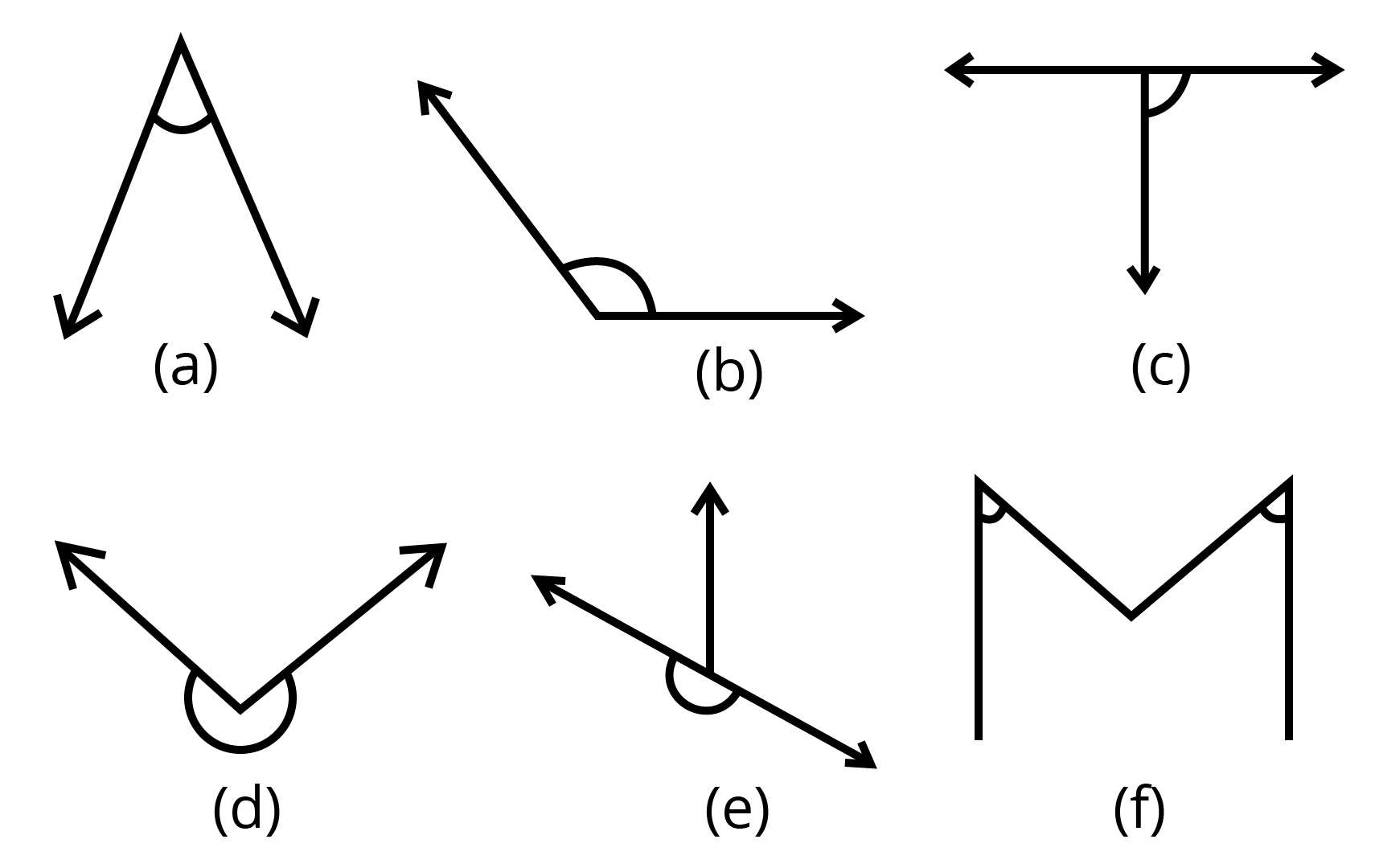उत्तर:

1. न्यून कोण

2. अधिक कोण

3. समकोण

4. प्रतिवर्ती कोण

5. ऋजुकोण

6. न्यून कोण

### प्रश्नावली 5.4

1. निम्न के क्या माप हैं:

1. एक समकोण

2. एक ऋजुकोण

उत्तर: $~\left( a \right)\text{ }90{}^\circ \text{ }\left( b \right)\text{ }180{}^\circ$

2. बताइए सत्य (T) या असत्य (F):

1. एक न्यून कोण का माप $< 90^\circ$ है।

2. एक अधिक कोण का माप $< 90^\circ$ है।

3. एक प्रतिवर्ती कोण का माप $> 180^\circ$ है।

4. एक सम्पूर्ण घूर्णन का माप $360^\circ$ है।

5. यदि $m\angle A\text{ }=\text{ }53$ और $m\angle B\text{ }=\text{ }35$ है, तो $m\angle A\text{ }>\text{ }m\angle B$ है।

उत्तर:

1. T

2. F

3. T

4. T

5. T

3. निम्न के माप लिखिए:

1. कुछ निम्न कोण

2. कुछ अधिक कोण

(प्रत्येक के दो उदाहरण दीजिए)

उत्तर: $\left( a \right)\text{ }60{}^\circ ,\text{ }45{}^\circ \text{ }\left( b \right)\text{ }120{}^\circ ,\text{ }135{}^\circ$

4. निम्न कोणों को चाँदे से मापिए और उनके नाम लिखिए: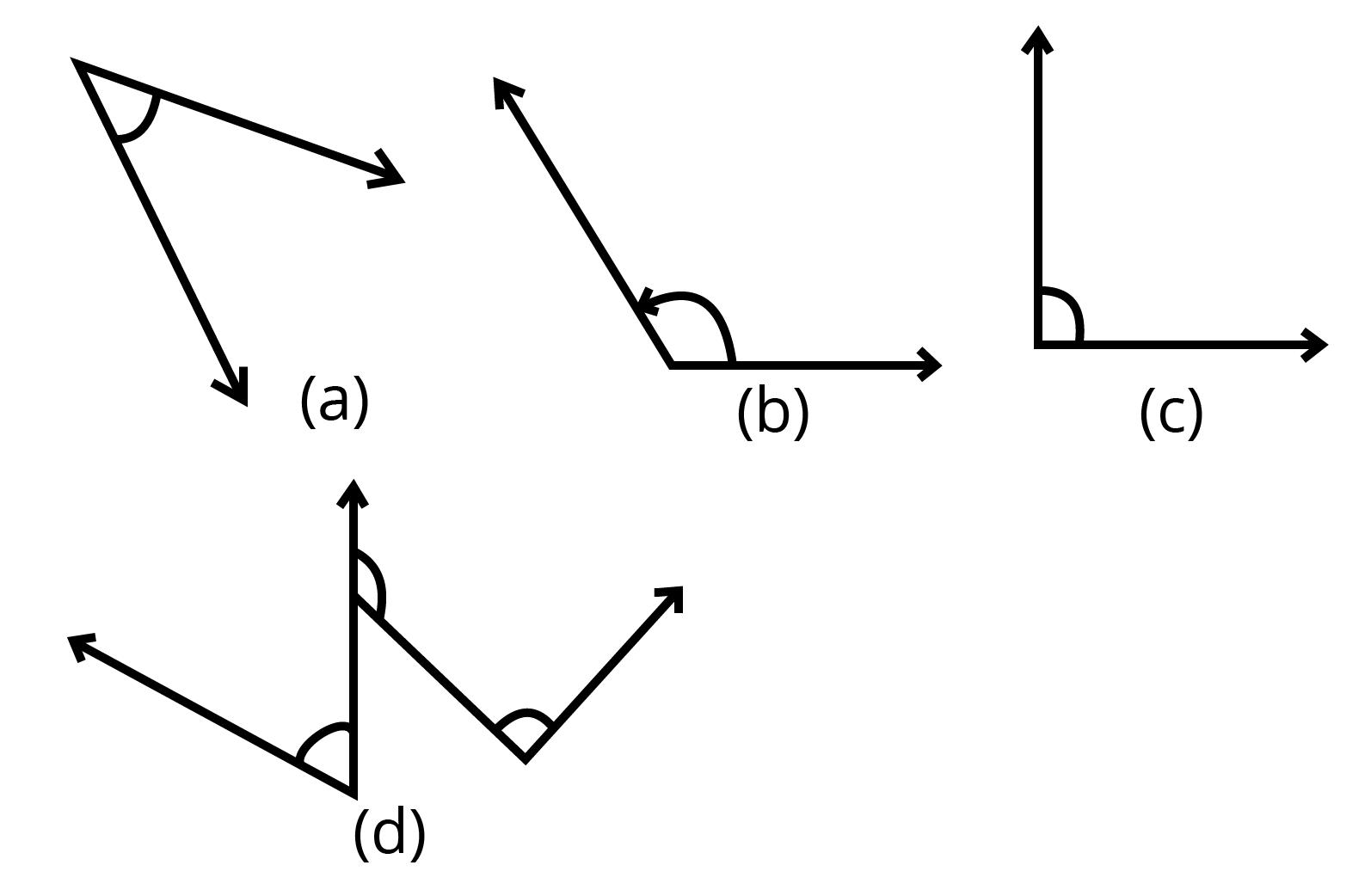उत्तर: (a) ${{40}^{0}}$ न्यून कोण

(b) ${{120}^{0}}$ अधिक कोण

(c) ${{90}^{0}}$ समकोण

(d) बायें से दायें: पहला कोण (समकोण) $={{60}^{0}}$,

दूसरा कोण (अधिक कोण$)=135{{~}^{0}}$

तीसरा कोण (न्यून कोण) $=90{{~}^{0}}$

5. किस कोण का माप बड़ा है?
पहले आकलन (estimate) कीजिए फिर मापिए।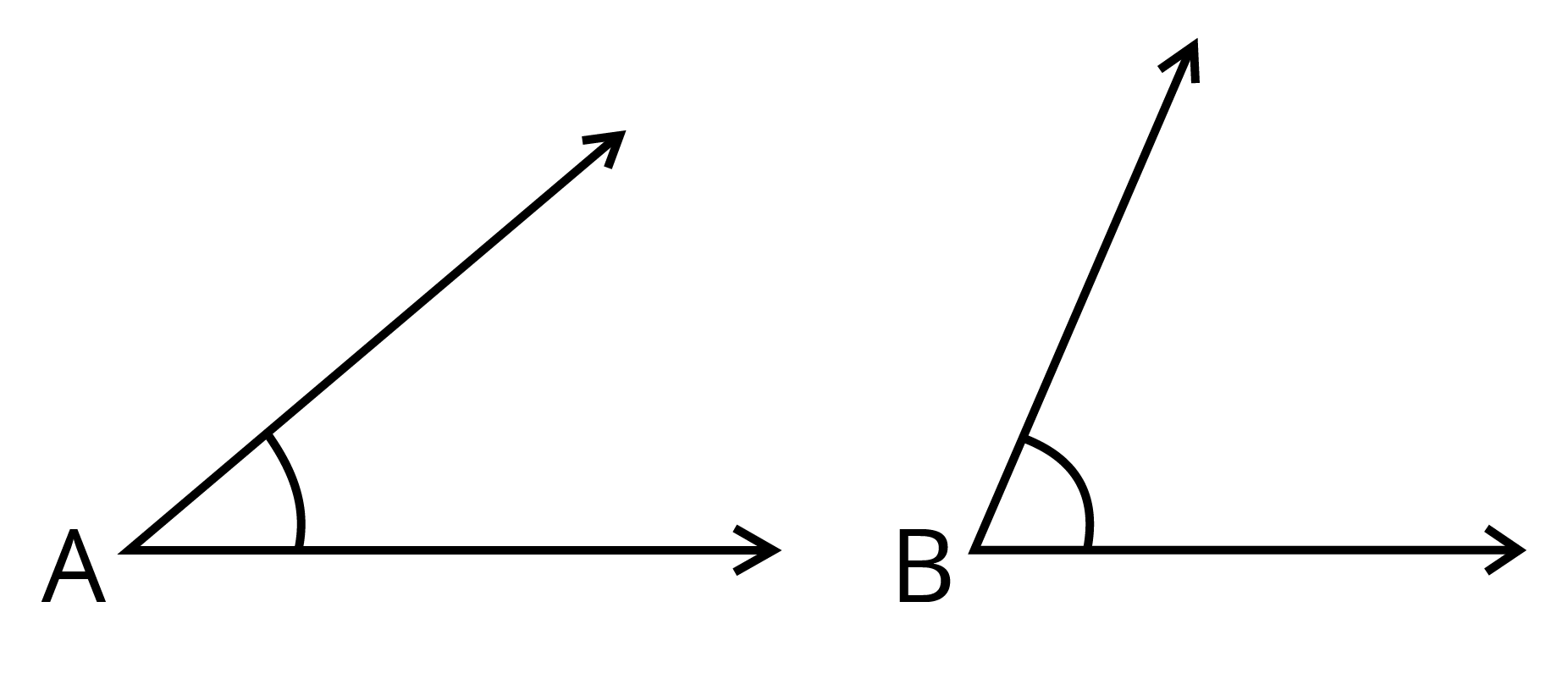उत्तर: $\angle B\left( {{65}^{0}} \right)\text{ }>\angle A\left( {{40}^{0}} \right)$

6. निम्न दो कोणों में से किस कोण का माप बड़ा है? पहले आकलन कीजिए और मापन द्वारा पुष्टि कीजिए।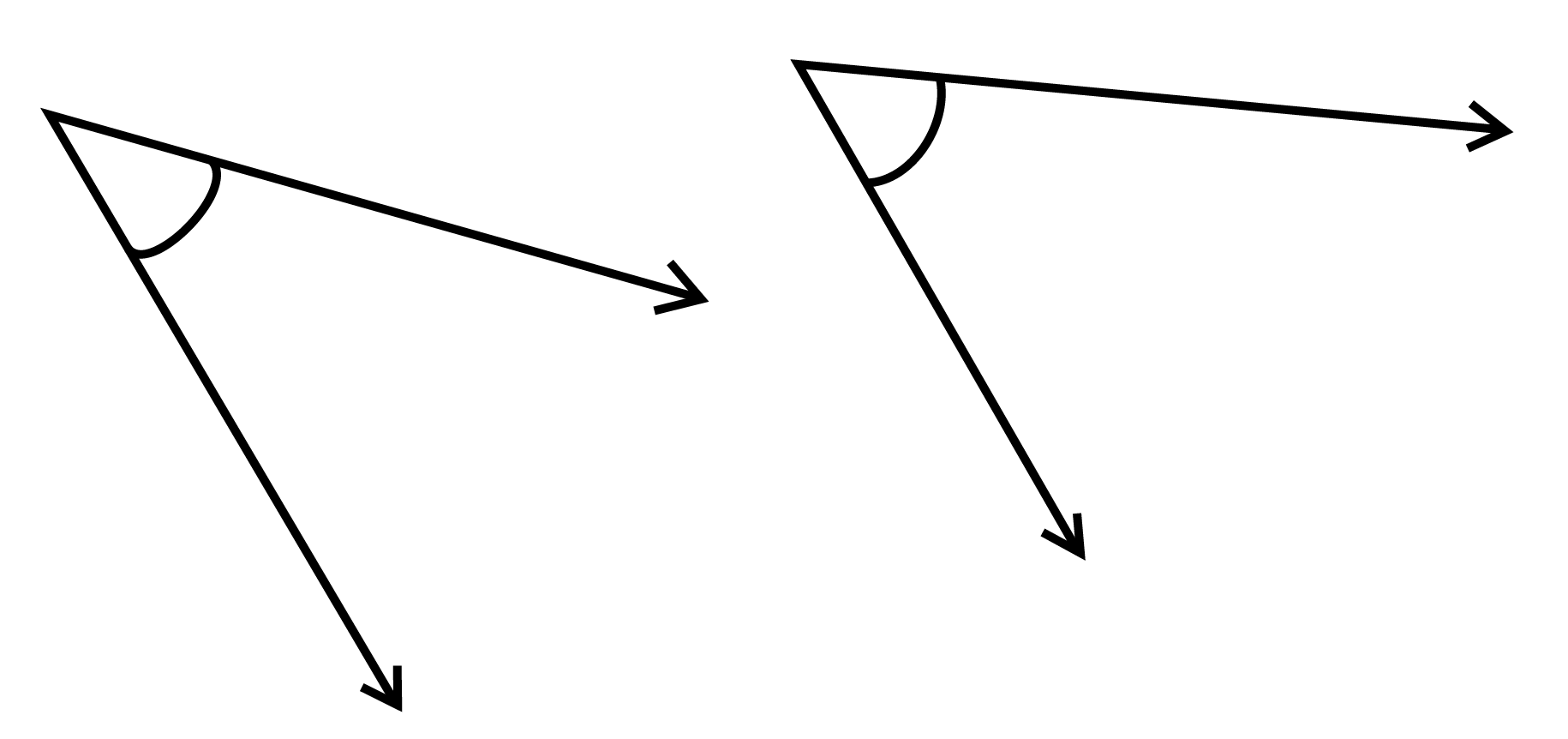उत्तर: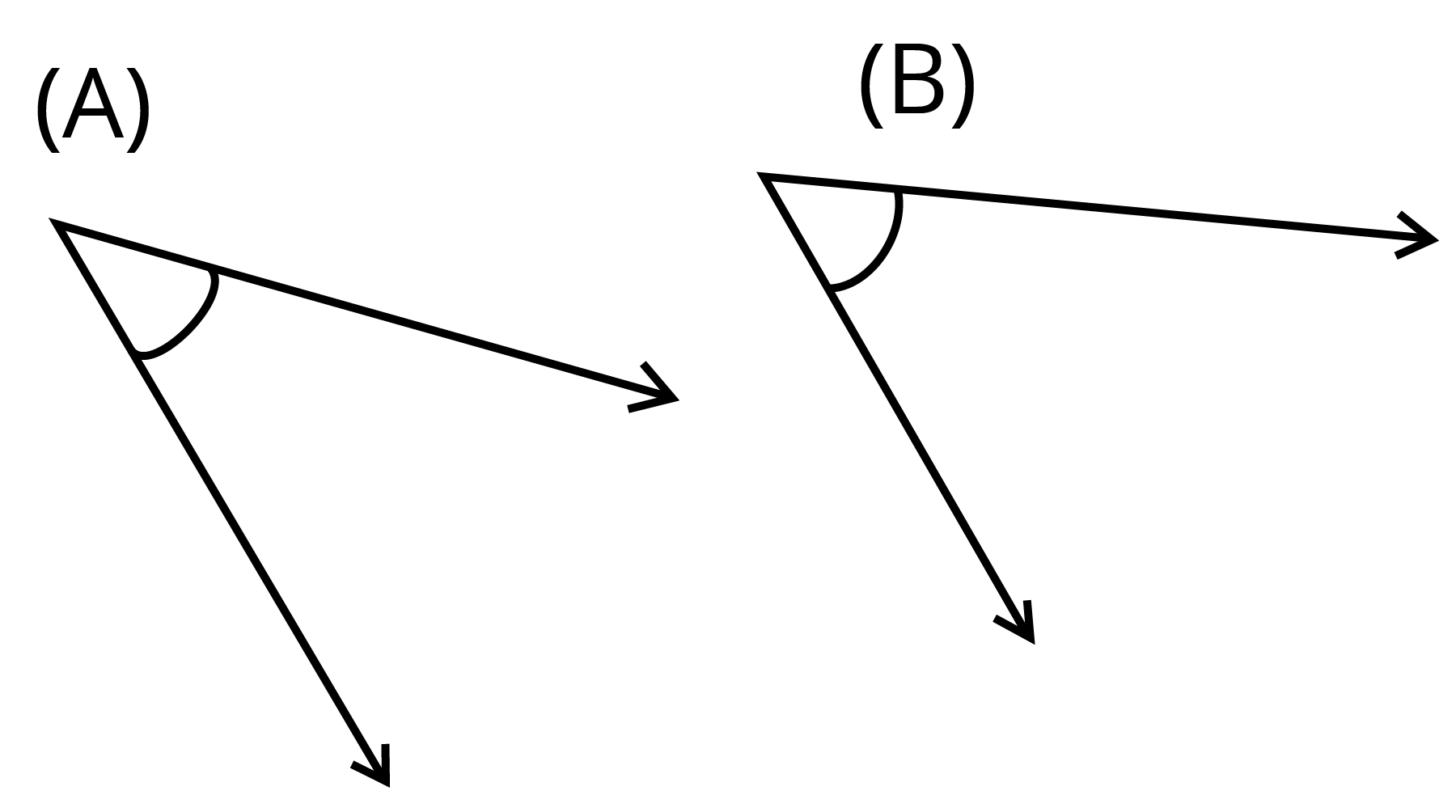चित्र (A)को मापने पर ${{40}^{0}}$ का कोण है।

चित्र (B) को मापने पर ${{60}^{0}}$ का कोण है।

बाद वाला (A) कोण बड़ा है।

7. निम्न कोण, अधिक कोण, समकोण और ऋजुकोण से रिक्त स्थानों को भरिए

1. वह कोण, जिसका माप एक समकोण के माप से कम है,             होता है।

2. वह कोण, जिसका माप एक समकोण के माप से अधिक है,           होता है।

3. वह कोण, जिसका माप दो समकोणों के योग के बराबर है,            होता है।

4. यदि दो कोणों के मापों का योग समकोण के माप के बराबर है, तो प्रत्येक कोण            होता है।

5. यदि दो कोणों के मापों का योग एक ऋजुकोण के माप के बराबर है, और इनमें से एक कोण न्यूनकोण है, तो दूसरा कोण              होना चाहिए।

उत्तर:

1. न्यूनकोण

2. अधिककोण

3. ऋजुकोण

4. न्यूनकोण

5. अधिककोण

8. नीचे दी आकृति में दिए प्रत्येक कोण का माप ज्ञात कीजिए (पहले देखकर आकलन कीजिए और फिर चाँदे से मापिए।):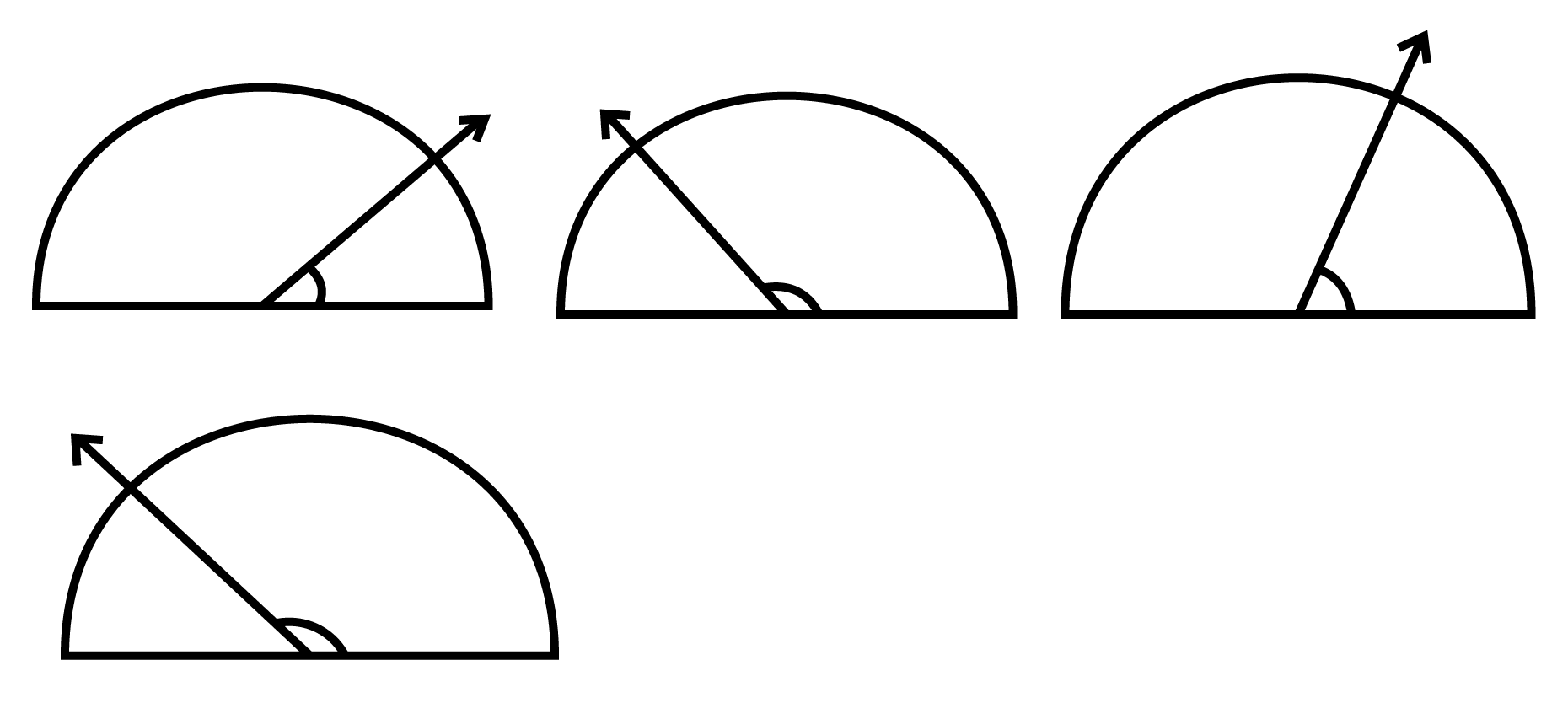उत्तर: आकलन करने पर (क्रमश:) $40{}^\circ ,\text{ }110{}^\circ ,\text{ }70{}^\circ ,\text{ }130{}^\circ$

चांदे से मापने पर (क्रमशः) $30{}^\circ ,\text{ }120{}^\circ ,\text{ }60{}^\circ ,\text{ }150{}^\circ$

9. नीचे दी आकृति में घड़ी की सुइयों की बीच के कोण का माप ज्ञात कीजिए: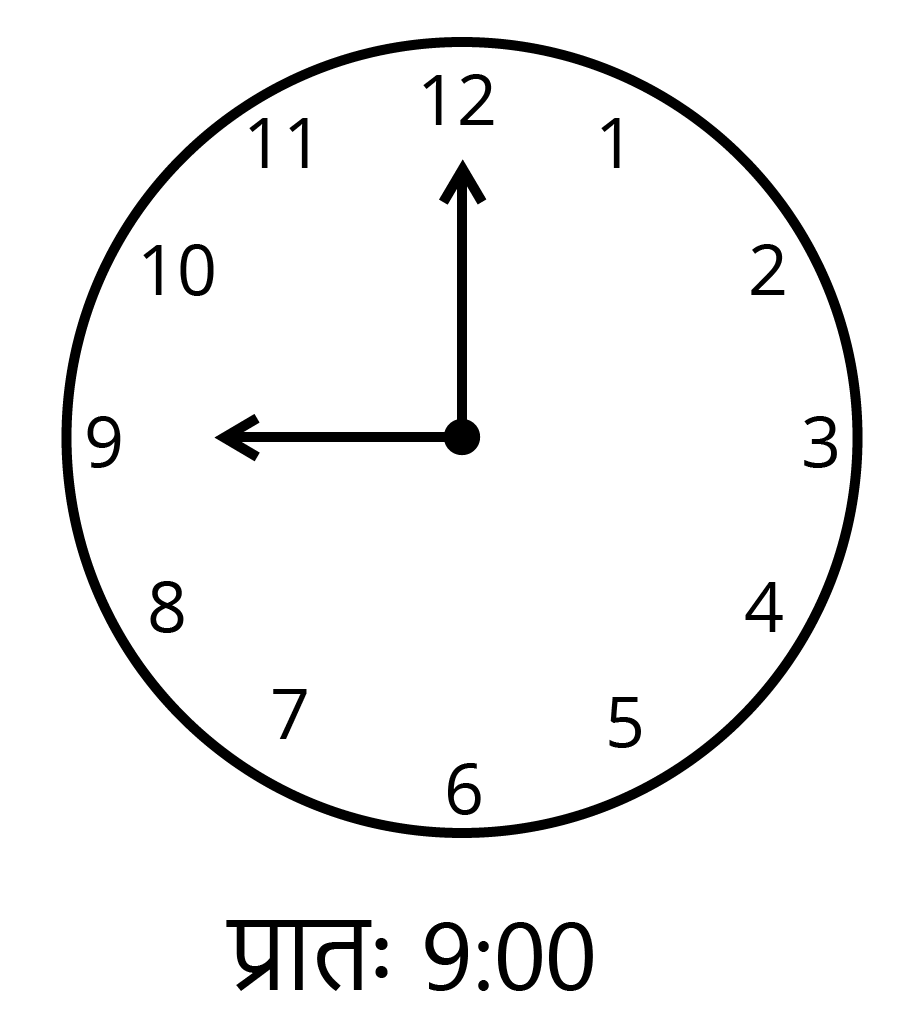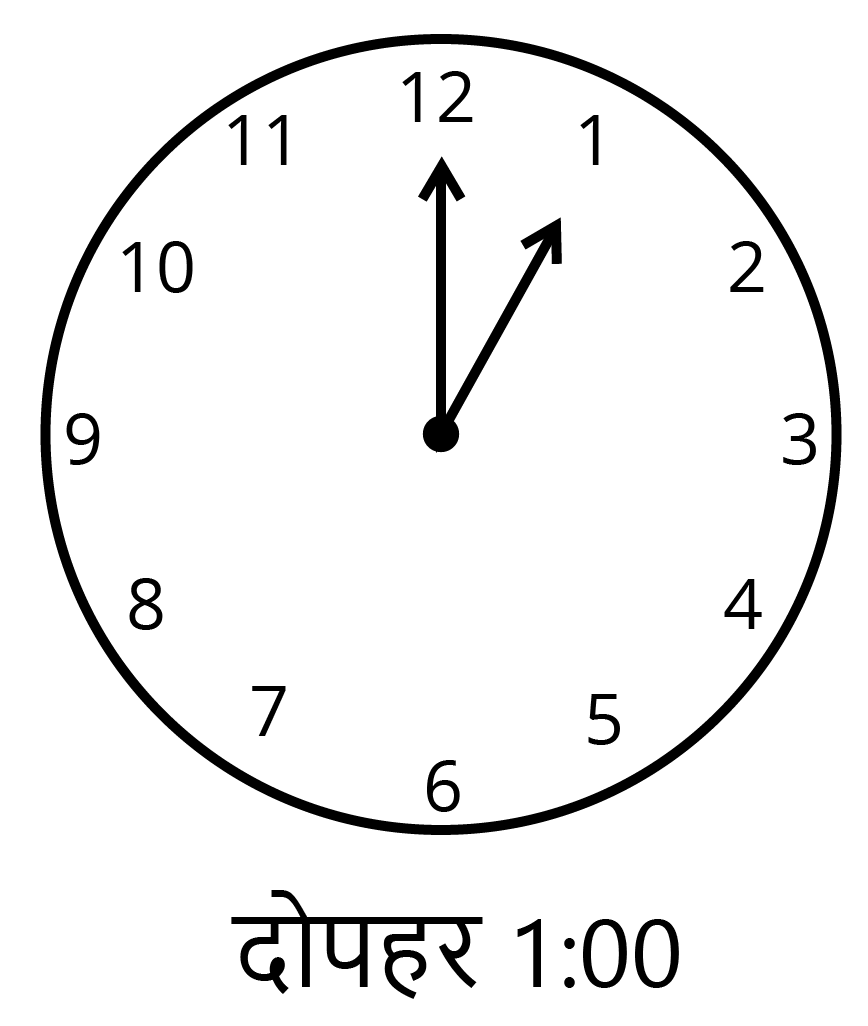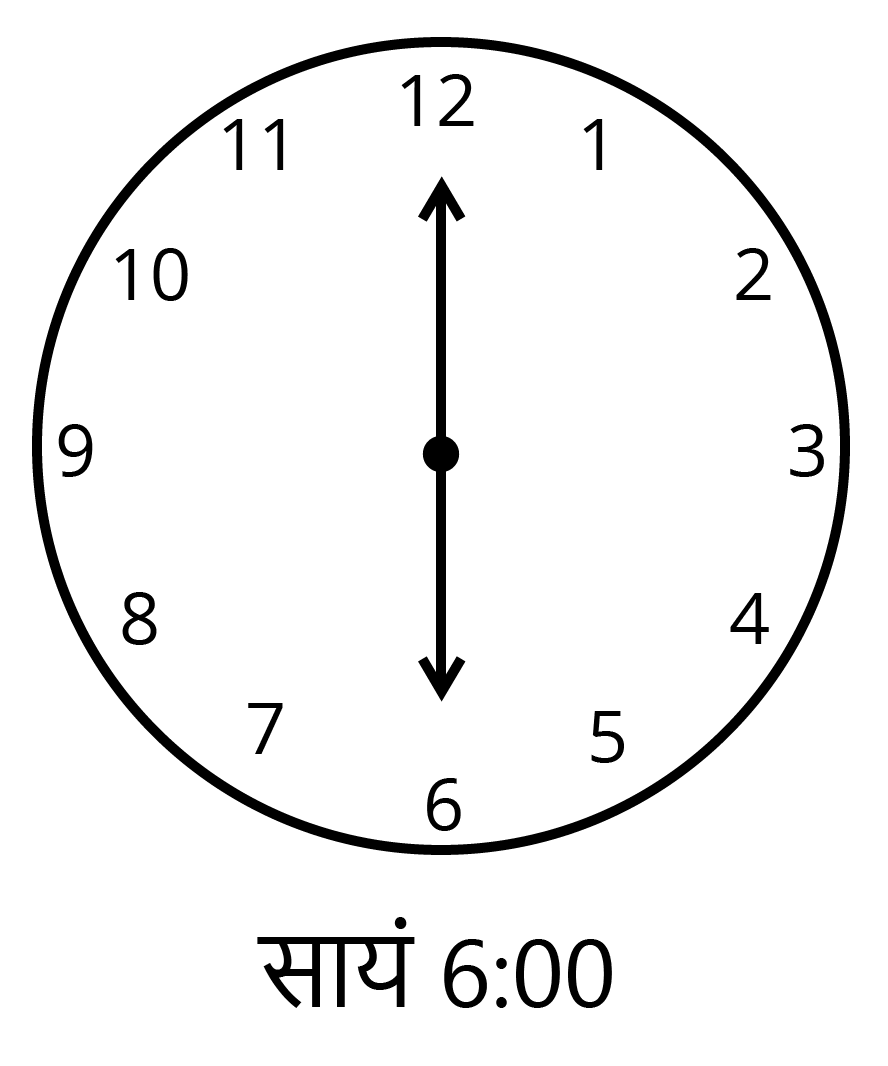उत्तर: $90{}^\circ ,\text{ }30{}^\circ ,\text{ }180{}^\circ$

10. खोज कीजिए:
दी हुई आकृति में चाँदा $30^\circ$ दर्शा रहा है। इसी आकृति को एक आवर्धन शीशे (magnifying glass) द्वारा देखिए।

1. क्या यह कोण बड़ा हो जाता है?

2. क्या कोण का माप बड़ा हो जाता है?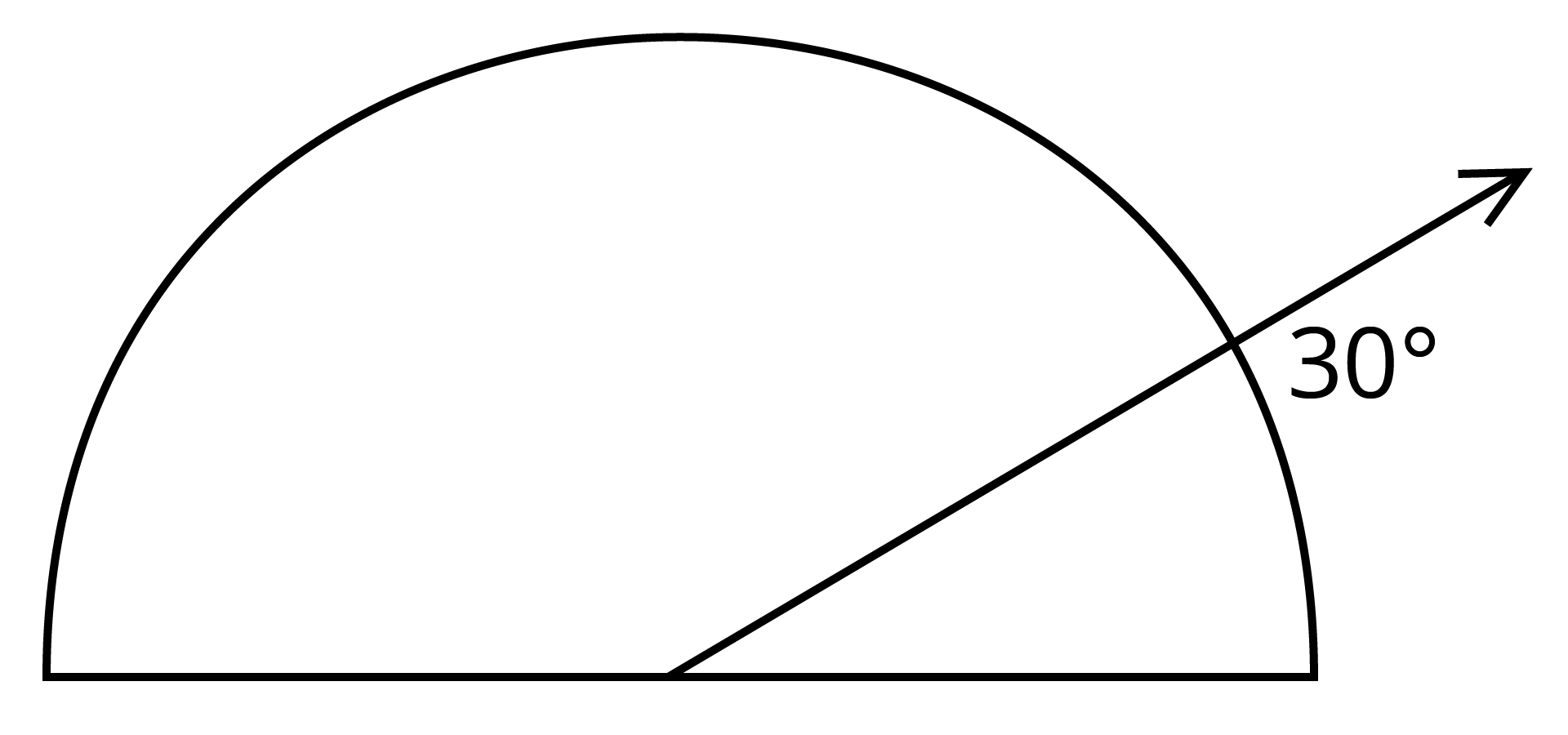उत्तर: आवर्धन शीशे में देखने पर भी कोण का माप नहीं बदलता है।

11. मापिए और प्रत्येक कोण को वर्गीकृत कीजिए: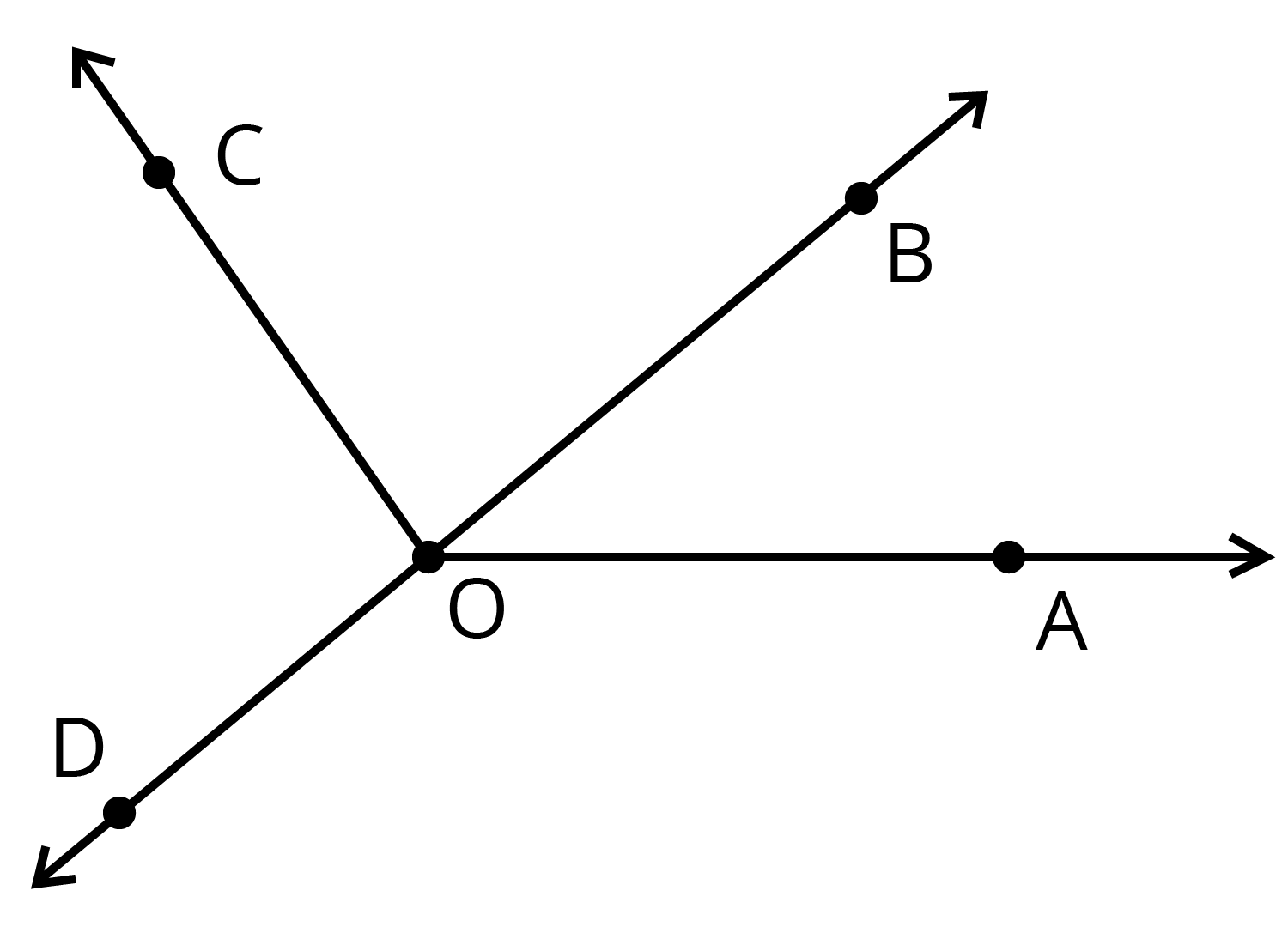उत्तर-

 कोण AOB AOC BOC DOC DOA DOB माप $40^\circ$ $125^\circ$ $85^\circ$ $95^\circ$ $140^\circ$ $180^\circ$ प्रकार न्यून कोण प्रतिवर्ती कोण न्यून कोण अधिक कोण प्रतिवर्ती कोण ऋजु कोण

### प्रश्नावली 5.5

1. निम्नलिखित में से कौन लंब रेखाओं का उदाहरण हैं?

1. मेज़ के ऊपरी सिरे की आसन्न भुजाएँ

2. रेल पथ की पटरियाँ

3. अक्षर $L$ बनाने वाले रेखाखंड

4. अक्षर $V$ बनाने वाले रेखाखंड

उत्तर: (a) मेज़ के ऊपरी सिरे की आसन्न भुजाएँ

और (c) अक्षर $L$ बनाने वाले रेखाखंड

2. मान लिजिए रेखाखंड $\mathbf{PQ}$ रेखाखंड $\mathbf{XY}$ पर लंब है। मान लिजिए ये परस्पर बिंदु $\mathbf{A}$ पर प्रतिच्छेद करते हैं। कोण $\mathbf{PAY}$ की माप क्या है?

उत्तर: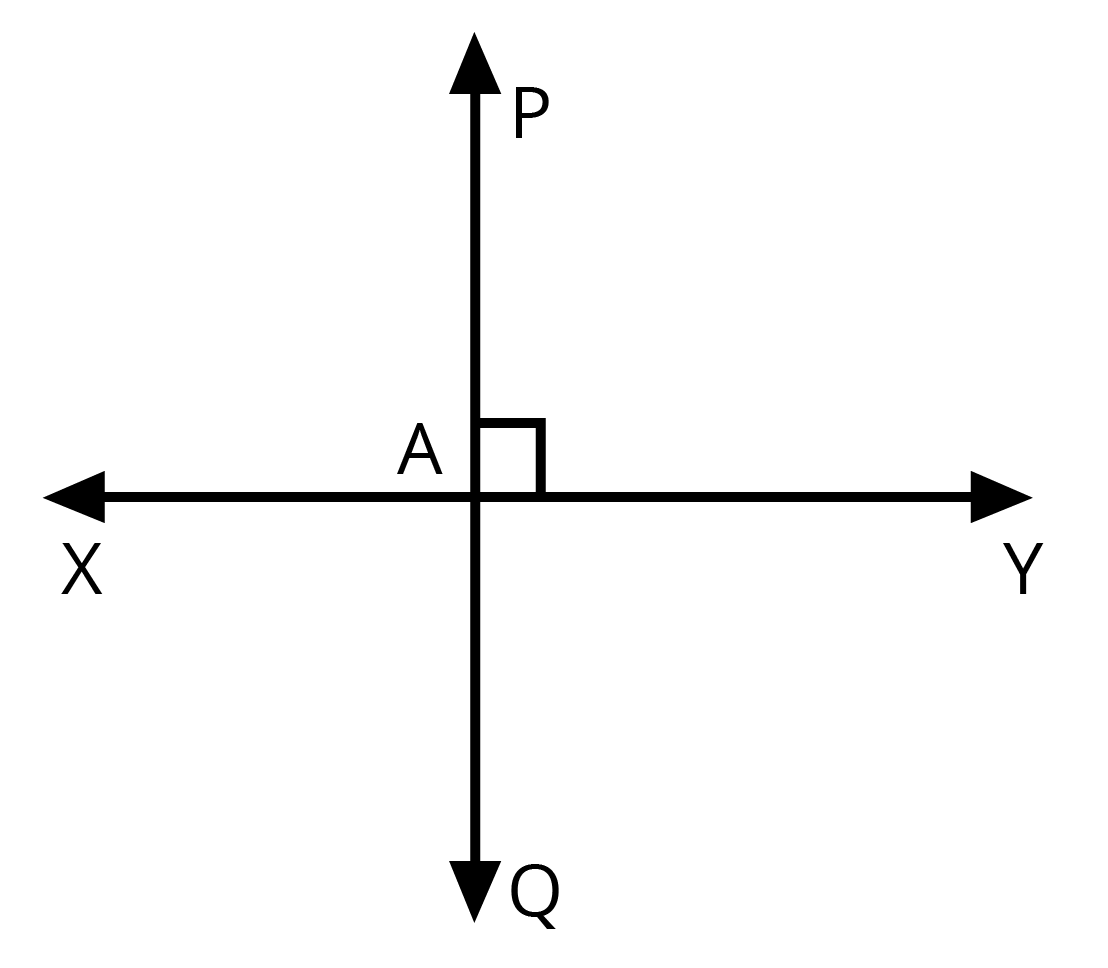$PQ\bot XY$

$\angle PAY={{90}^{0}}$

3. आपके ज्यामिति बॉक्स में दो सेट स्क्वेयर हैं। इनके कोनों पर बने कोणों के माप क्या हैं? क्या इनमें से कोई ऐसी माप है जो दोनों में उभयनिष्ट है?

उत्तर:

दोनों ही सेट स्क्वेयर समकोण त्रिभुज हैं। इसलिए दोनों में उभयनिष्ठ कोण की माप 90° है। एक सेट स्क्वेयर के बाकी दोनों कोण 45° माप के हैं। दूसरे सेट स्क्वेयर के बाकी कोणों की माप 30° और 60° हैं।

4. इस आकृति को ध्यान से देखिए। रेखा $\mathbf{m}$ पर लंब है।

1. क्या $\mathbf{CE}\text{ }=\text{ }\mathbf{EG}$ है।

2. क्या रेखा $\mathbf{PE}$ रेखाखंड $\mathbf{CG}$ को समद्विभाजित करती है।

3. कोई दो रेखाखंड के नाम लिखिए जिनके लिए $\mathbf{PE}$ लंब समद्विभाजक है।

4. क्या निम्नलिखित सत्य हैं?

1. $\mathbf{AC}\text{ }>\text{ }\mathbf{FG}$

2. $\mathbf{CD}\text{ }=\text{ }\mathbf{GH}$

3. $\mathbf{BC}\text{ }<\text{ }\mathbf{EH}$

उत्तर:

1. हाँ $\mathbf{CE}\text{ }=\text{ }\mathbf{EG}$

2. हाँ, रेखा $\mathbf{PE}$ रेखाखंड $\mathbf{CG}$ को समद्विभाजित करती है।

3. दो रेखाखंड $BH$ और $CG$, जिनके लिए $\mathbf{PE}$ लंब समद्विभाजक है।

4. सभी सत्य हैं।

### प्रश्नावली 5.6

1.निम्नलिखित त्रिभुजों के प्रकार लिखिए:

1. त्रिभुज जिसकी भुजाएँ $7$ सेमी, $8$ सेमी और $9$ सेमी हैं।

उत्तर: विषमबाहु त्रिभुज

1. $\Delta \text{ }ABC$ जिसमें $AB\text{ }=\text{ }8.7$ सेमी, $AC\text{ }=\text{ }7$ सेमी और $BC\text{ }=\text{ }6$ सेमी है।

उत्तर:विषमबाहु त्रिभुज

1. $\Delta \text{ }PQR$ जिसमें $PQ\text{ }=\text{ }QR\text{ }=\text{ }PR\text{ }=\text{ }5$ सेमी है।

उत्तर:समबाहु त्रिभुज

1. $\Delta \text{ }DEF$ जिसमें $m\angle D\text{ }=\text{ }90$ है।

उत्तर: समकोण त्रिभुज

1. $\Delta \text{ }XYZ$ जिसमें $m\angle Y\text{ }=\text{ }90$ और $XY\text{ }=\text{ }YZ$ है।

उत्तर:समद्विबाहु समकोण त्रिभुज

1. $\Delta \text{ }LMN$ जिसमें $m\angle L\text{ }=\text{ }30,\text{ }m\angle M\text{ }=\text{ }70$ और $m\angle N\text{ }=\text{ }80$ है।

उत्तर:  न्यूनकोण त्रिभुज

2. निम्न का सुमेलन कीजिए:

 त्रिभुज की माप त्रिभुज के प्रकार (i) समान लंबाई की तीन भुजाएँ (a) विषमबाहु समकोण त्रिभुज (ii) समान लंबाई की दो भुजाएँ (b) समद्विबाहु समकोण त्रिभुज (iii) अलग-अलग लंबाईयों की सभी भुजाएँ (c) अधिक कोण त्रिभुज (iv) $3$ न्यूनकोण (d) समकोण त्रिभुज (v) $1$ समकोण (e) समबाहु त्रिभुज (vi) $1$ अधिक कोण (f) न्यून कोण त्रिभुज (vii) दो बराबर लंबाईयों की भुजाओं के साथ एक समकोण (g) समद्विबाहु त्रिभुज

उत्तर: $\left( i \right)\text{ }e,\left( ii \right)\text{ }g,\left( iii \right)\text{ }a,\left( iv \right)\text{ }f,\left( v \right)\text{ }d,\left( vi \right)\text{ }c,\left( vii \right)\text{ }b$

3. निम्नलिखित त्रिभुजों में से प्रत्येक का दो प्रकार से नामकरण कीजिए (आप कोण का प्रकार केवल देखकर ज्ञात कर सकते हैं।)

उत्तर:

1. समद्विबाहु और न्यूनकोण त्रिभुज

2.  विषमबाहु और समकोण त्रिभुज

3. समद्विबाहु और अधिककोण त्रिभुज

4. समद्विबाहु और समकोण त्रिभुज

5. समबाहु और न्यूनकोण त्रिभुज

6. विषमबाहु और अधिककोण त्रिभुज

4. माचिस की तीलियों की सहायता से त्रिभुज बनाने का प्रयत्न कीजिए। इनमें से कुछ आकृति में दिखाए गए हैं। क्या आप निम्न से त्रिभुज बना सकते हैं?

1. 3 माचिस की तीलियाँ

2. 4 माचिस की तीलियाँ

3. 5 माचिस की तीलियाँ

4. 6 माचिस की तीलियाँ

उत्तर: $a,\text{ }c$ और $d$ की दशा (स्थिति) में त्रिभुज बन सकते हैं।

लेकिन $b$ की दशा में त्रिभुज नहीं बन सकता है।

### प्रश्नावली 5.7

1. सत्य (T) या असत्य (F) बताइये :

1. आयत का प्रत्येक कोण समकोण होता है।

2. आयत की सम्मुख भुजाओं की लंबाई बराबर होती है।

3. वर्ग के विकर्ण एक-दूसरे पर लंब होते हैं।

4. समचतुर्भुज की सभी भुजाएँ बराबर लंबाई की होती है।

5. समांतर चतुर्भुज की सभी भुजाएँ बराबर लंबाई की होती हैं।

6. समलंब की सम्मुख भुजाएँ समांतर होती है।

उत्तर:

1. सत्य

2. सत्य

3. सत्य

4. सत्य

5. असत्य

6. असत्य

2. निम्नलिखित के लिए कारण दीजिए:

1. वर्ग को एक विशेष प्रकार का आयत समझा जा सकता है।

उत्तर: जब किसी आयत की सभी भुजाएँ बराबर होती हैं तो उसे वर्ग कहते हैं।

1. आयत को एक विशेष प्रकार का समांतर चतुर्भुज समझा जा सकता है।

उत्तर: जब किसी समांतर चतुर्भुज का प्रत्येक कोण समकोण होता है तो उसे आयत कहते हैं।

1. वर्ग को एक विशेष प्रकार का समचतुर्भुज समझा जा सकता है।

उत्तर: जब किसी समचतुर्भुज के सभी कोण समकोण होते हैं तो उसे वर्ग कहते हैं।

1. वर्ग, आयत, समांतर चतुर्भुज और समचतुर्भुज में से प्रत्येक एक चतुर्भुज भी है।

उत्तर: इन सभी में भी चार भुजाएँ हैं।

1. वर्ग एक समांतर चतुर्भुज भी है।

उत्तर: वर्ग की सम्मुख भुजाएँ समांतर होती हैं।

3. एक समबहुभुज (regular) होता है, यदि उसकी सभी भुजाएँ बराबर हों और सभी कोण बराबर हो। क्या आप एक समचतुर्भुज (regular quadrilateral) की पहचान कर सकते है?

उत्तर: वर्ग को एक सम चतुर्भुज मान सकते है।

### प्रश्नावली 5.8

1. जाँच कीजिए कि निम्न में से कौन-सी आकृतियाँ बहुभुज है। यदि इनमें से कोई बहुभुज नहीं है, तो कारण बताइए।

उत्तर:

1. बंद आकृति नहीं है इसलिए बहुभुज नहीं है।

2. यह बहुभुज है।

3. रेखाखंडों से नहीं बना है इसलिए बहुभुज नहीं हैं।

4. पूर्णरूप से रेखाखंडों से नहीं बना है इसलिए बहुभुज नहीं है।

2. प्रत्येक बहुभुज का नाम लिखिए।

उत्तर:

1. चतुर्भुज

2. त्रिभुज

3. पंचभुज

4. अष्ट भुज

3. एक सम षड्‌भुज (regular hexagon) का एक रफ़ चित्र खींचिए। उसके किसी तीन शीर्षों को जोड़कर एक त्रिभुज बनाइए। पहचानिए कि आपने किस प्रकार का त्रिभुज खींचा है।

उत्तर: समबाहु त्रिभुज

4. एक सम अष्टभुज (regular octagon) का एक रफ़ चित्र खींचिए। [ यदि आप चाहें, तो वर्गांकित कागज़ (squared paper) का इस्तेमाल कर सकते हैं। इस अष्टभुज के चार शीर्षों को जोड़कर एक आयत खींचिए।

उत्तर:

5. किसी बहुभुज का विकर्ण उसके किन्हीं दो शीर्षों (आसन्न शीर्षों को छोड़कर) को जोड़ने से प्राप्त होता है (यह इसकी भुजाएँ नहीं होता है)। एक पंचभुज का रफ़ चित्र खींचिए और उसके विकर्ण खींचिए।

उत्तर:

### प्रश्नावली 5.9

1. निम्न का सुमेल कीजिए:

 (a) शंकु (image will be uploaded soon) (b) गोला (image will be uploaded soon) (c) बेलन (image will be uploaded soon) (d) घनाभ (image will be uploaded soon) (e)पिरामिड (image will be uploaded soon)

उत्तर: $\left( a \right){\rm{ }}ii,\,\,\,\left( b \right){\rm{ }}iv,\,\,\,\left( c \right){\rm{ }}v,\,\,\,\left( d \right){\rm{ }}iii,\,\,\,\left( e \right){\rm{ }}i$

2. निम्न किस आकार के हैं?

1. आपका ज्यामिति बॉक्स

2. एक ईंट

3. एक माचिस की डिब्बी

4. सड़क बनाने वाला रोलर(roller)

5. एक लड्डू

उत्तर:

1. घनाभ

2. घनाभ

3. घनाभ

4. बेलन

5. गोला

### NCERT Solutions for Class 6 Maths Chapter 5 Understanding Elementary Shapes in Hindi

Chapter-wise NCERT Solutions are provided everywhere on the internet with an aim to help the students to gain a comprehensive understanding. Class 6 Maths Chapter 5 solution Hindi medium is created by our in-house experts keeping the understanding ability of all types of candidates in mind. NCERT textbooks and solutions are built to give a strong foundation to every concept. These NCERT Solutions for Class 6 Maths Chapter 5 in Hindi ensure a smooth understanding of all the concepts including the advanced concepts covered in the textbook.

NCERT Solutions in Hindi medium have been created keeping those students in mind who are studying in a Hindi medium school. These NCERT Solutions for Class 6 Maths Understanding Elementary Shapes in Hindi medium pdf download have innumerable benefits as these are created in simple and easy-to-understand language. The best feature of these solutions is a free download option. Students of Class 6 can download these solutions at any time as per their convenience for self-study purpose.

These solutions are nothing but a compilation of all the answers to the questions of the textbook exercises. The answers/solutions are given in a stepwise format and very well researched by the subject matter experts who have relevant experience in this field. Relevant diagrams, graphs, illustrations are provided along with the answers wherever required. In nutshell, NCERT Solutions for Class 6 Maths in Hindi come really handy in exam preparation and quick revision as well prior to the final examinations.

## FAQs on NCERT Solutions for Class 6 Maths Chapter 5 - In Hindi

1. Can Hindi Medium students make use of Vedantu’s NCERT Solutions for Class 6 Mathematics?

Vedantu aims at producing high-caliber intellectuals all across India. Vedantu goes the extra mile in preparing its Mathematical solutions for Class 6 in the Hindi language as well. Students from both English and Hindi medium schools have the opportunity to access Vedantu’s NCERT Solutions. The solutions in Hindi are prepared by expert Mathematics teachers who have experience with Hindi medium textbooks. These solutions are available free of cost. Hence the answers are reliable and easy to use for Hindi medium students.

2. Which chapters in NCERT Solutions for Class 6 Maths require more practice?

Mathematics is a subject that requires consistent and regular practice. Students should not take any chapter lightly and practice all chapters with equal diligence.  Class 6 Mathematics syllabus lays the foundation of important mathematical concepts for the upcoming academic years. Hence, students should do their best to understand the concepts explained in the NCERT textbook and practice them well. They can always refer to NCERT's solutions and extra reading material.

3. What are the key features of NCERT Solutions for Class 6 Maths?

Vedantu's NCERT Solutions are prepared after keeping in mind the guidelines from the CBSE. They are curated by experienced mathematics teachers who have worked in their field of expertise for several years. The solutions are explained in a pretty straightforward way with the help of relevant figures wherever necessary. An exceptional key feature of Vedantu is that it provides Mathematical solutions for students enrolled in English as well as Hindi medium schools. These solutions can be accessed on Vedantu’s website (vedantu.com). Students can also download the app of Vedantu from Google Playstore and download offline solutions in PDF format.

4. Define angles and their types covered under NCERT Solutions for Class 6 Maths Chapter 5?

According to Chapter 5 of Class 6 Mathematics, an angle is a figure formed by two rays. Different types of angles are as follows:

• Right angle: An angle of exactly 90 degrees.

• Straight angle: An angle of exactly 180 degrees.

• Acute angle: An angle that measures less than 90 degrees

• Obtuse angle: An angle that measures more than 90 degrees and less than 180 degrees.

• Reflex angle: An angle more than 180 degrees but less than 360 degrees.

5. Explain solid shapes covered under NCERT Solutions for Class 6 Maths?

Class 6 Chapter 5, "Understanding Elementary Shapes," introduces students to fundamental concepts related to shapes and different kinds of shapes along with their properties. Three-dimensional shapes are also called solid shapes as these shapes are not "flat shapes." Shapes such as cuboid, cube, cone, cylinder, sphere, and pyramid are examples of such shapes. These shapes are characterized by faces, edges, vertices.